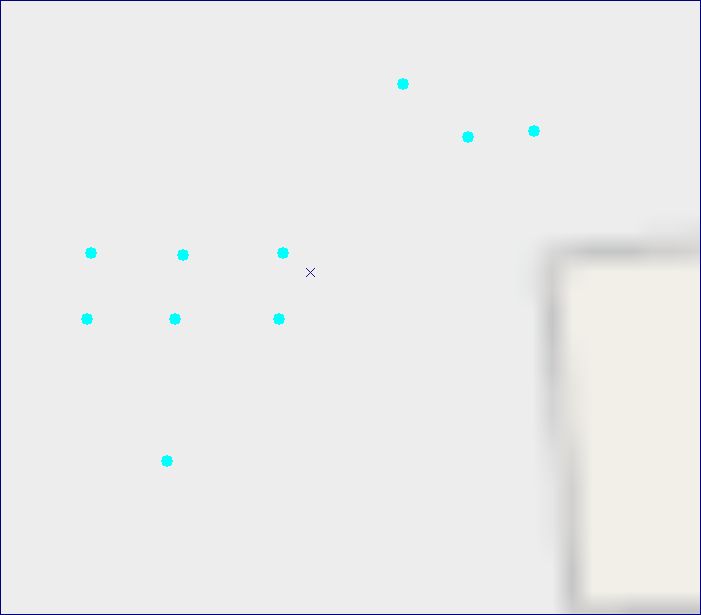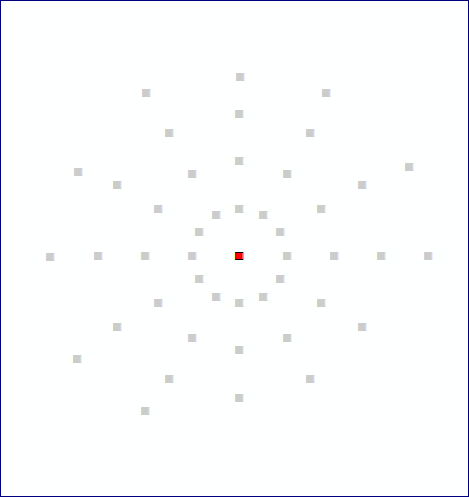# How to arrange points by circle or by star?

709
7
02-01-2017 12:34 AMNew Contributor

ArcGIS 10.17 RepliesbyMVP Esteemed Contributor

could you elaborate?

Do you want to sort those points by angle?

Do you want to move those points on to a circle?

What developmenet language (python etc)

Given those points, you may as well create new points that are on a circle if that indeed represents your attempt to create points on a circle.New Contributor

I want like thatbyMVP Esteemed Contributor

``def _circle(radius=100, clockwise=True, theta=1, rot=0.0, scale=1, xc=0.0, yc=0.0):    """Produce a circle/ellipse depending on parameters.    :  see https://github.com/Dan-Patterson/numpy_samples/tree/master/geometry/scripts    """    if clockwise:        angles = np.deg2rad(np.arange(180.0, -180.0-theta, step=-theta))    else:        angles = np.deg2rad(np.arange(-180.0, 180.0+theta, step=theta))    x_s = radius*np.cos(angles)            # X values    y_s = radius*np.sin(angles) * scale    # Y values    pnts = np.c_[x_s, y_s]    if rot != 0:        rot_mat = rot_matrix(angle=rot)        pnts = (np.dot(rot_mat, pnts.T)).T    pnts = pnts + [xc, yc]    return pnts‍‍‍‍‍‍‍‍‍‍‍‍‍‍‍‍``

which can be used to generate points at a desired angular spacing, radius and center... for example

``>>> ... c = _circle(radius=100, theta=15, xc=0, yc=0)>>> carray([[-100.000,  0.000],       [-96.593,  25.882],       [-86.603,  50.000],       [-70.711,  70.711],       [-50.000,  86.603],       [-25.882,  96.593],       [ 0.000,  100.000],       [ 25.882,  96.593],       [ 50.000,  86.603],       [ 70.711,  70.711],       [ 86.603,  50.000],       [ 96.593,  25.882],       [ 100.000,  0.000],       [ 96.593, -25.882],       [ 86.603, -50.000],       [ 70.711, -70.711],       [ 50.000, -86.603],       [ 25.882, -96.593],       [ 0.000, -100.000],       [-25.882, -96.593],       [-50.000, -86.603],       [-70.711, -70.711],       [-86.603, -50.000],       [-96.593, -25.882],       [-100.000, -0.000]])‍‍‍‍‍‍‍‍‍‍‍‍‍‍‍‍‍‍‍‍‍‍‍‍‍‍‍‍``New Contributor

I marked accidentally.

Where I have to paste this code?byMVP Esteemed Contributor

it is python... it is used as a function to generate the point coordinates as per your requirements.  The code generates one condition (ie circle center, radius and angular spacing of the values on the circle)

You would implement within a loop or similar collection structure to get all of them.

It is the foundation for the answer... I suspect you are looking for an already packaged solution.  You would be advised to specify what development environment you are using, what language you program in and where the results are going to goNew Contributor

I can't arrange it in simple steps?byMVP Esteemed Contributor

It might be useful to move this thread to the developer environment you are working in since the 'Developer' place is far too general

Hopefully this is for use within arcmap and not some other arc* software incarnation.